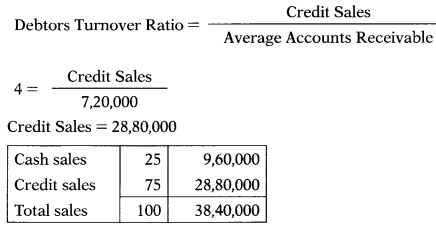# Ratio Analysis – Corporate and Management Accounting MCQ

Going through the Ratio Analysis – Corporate and Management Accounting CS Executive MCQ Questions with Answers you can quickly revise the concepts.

## Ratio Analysis – Corporate and Management Accounting MCQs

Question 1.
Which of the following assets is not a quick current asset for the purpose of calculating acid test ratio
(A) Short term bills receivables
(B) Cash
(C) Stock
(D) Debtors less provision for bad and doubtful debts
(C) Stock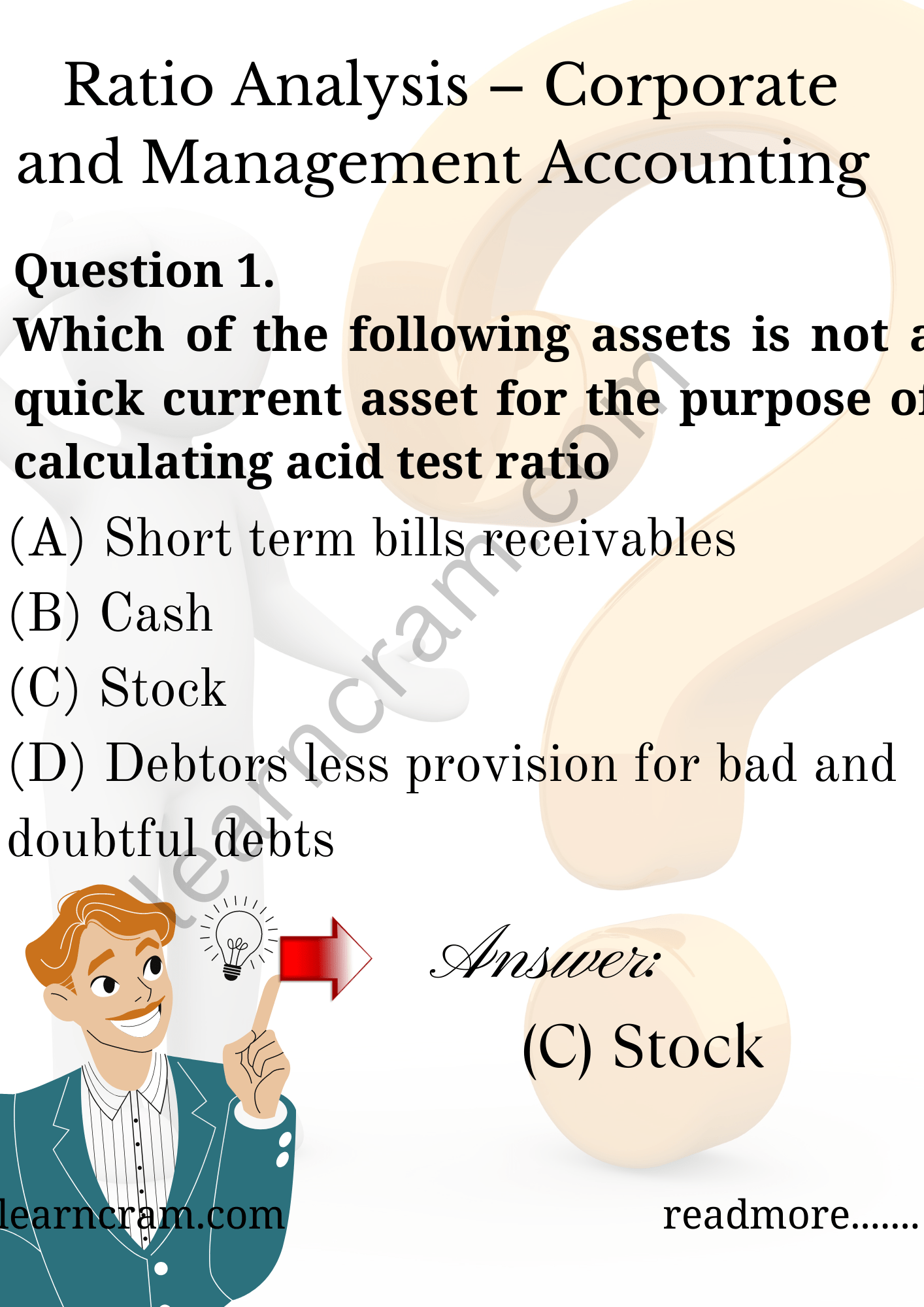Question 2.
Dividing net sales by average debtors would yield
(A) Acid test ratio
(B) Return on sales ratio
(C) Debtors turnover ratio
(D) Debtors velocity
(C) Debtors turnover ratio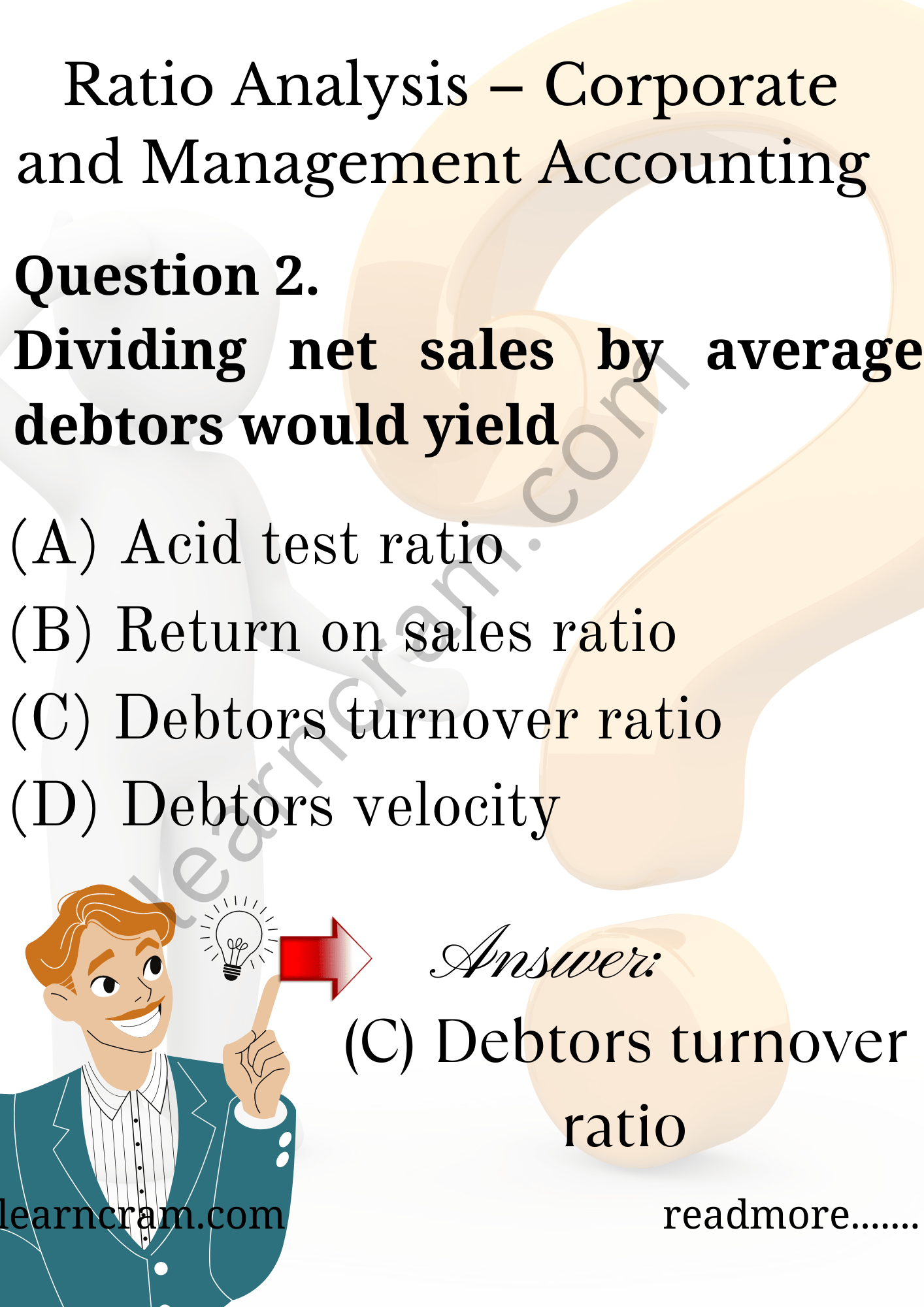Question 3.
Which of the following liabilities are taken into account for acid test ratio
2. Bank overdraft
3. Bills payable
4. Outstanding expenses
5. Redeemable debentures.
Select the correct answer from the options given below:
(A) 1,2, 3, 4 and 5
(B) 1,3 and 4
(C) 1,2, 3 and 4
(D) 1,3, 4 and 5
(B) 1,3 and 4

Question 4.
ROI – Return on investment is equal to:
(A) PAT/Tangible Net Worth
(B) PAT/Net Tangible Assets
(C) PAT/Paid up Capital
(D) Gross Profit/Gross Assets
(B) PAT/Net Tangible Assets

Question 5.
Capital gearing ratio is ………..
(A) Market test ratio
(B) Long-term solvency ratio
(C) Liquid ratio
(D) Turnover ratio
(B) Long-term solvency ratio

Question 6
…………… is also known as working capital ratio.
(A) Current ratio
(B) Quick ratio
(C) Liquid ratio
(D) Debt-equity ratio
(A) Current ratio

Question 7.
What ratio can be used to inefficient buying habits
(A) Inventory turnover ratio
(B) Gross margin ratio
(C) Equity multiplier
(D) Debt ratio
(A) Inventory turnover ratio

Question 8.
A ratio that compares investors’ and creditors’ stake in a company is
(A) Debt ratio
(B) Debt equity ratio
(C) Equity ratio
(D) Investor creditor ratio
(B) Debt equity ratio

Question 9.
The ratio that explains how efficiently companies use their assets to generate revenue is
(A) Revenue asset ratio
(B) Receivable turnover ratio
(C) Income ratio
(D) Asset turnover ratio
(D) Asset turnover ratio

Question 10.
What does the accounts receivable turnover ratio tell us
(A) How often account receivable received
(B) How many time account receivable is collected
(C) Account receivable balance at the end of the period
(D) Bad debt balance at the year end
(B) How many time account receivable is collected

Question 11.
The best ratio to evaluate short-term liquidity is:
(A) Working capital turnover ratio
(B) Current ratio
(C) Creditors velocity
(D) All of the above
(B) Current ratio

Question 12.
The DuPont Analysis uses the following ratios except:
(A) Debt ratio
(B) Profit margin
(C) Total asset turnover
(D) Financial leverage
(A) Debt ratio

Question 13.
Which best describes the gross margin ratio
(A) Leverage ratio
(B) Liquidity ratio
(C) Coverage ratio
(D) Profitability ratio
(D) Profitability ratio

Question 14.
Inventory turnover ratio evaluates:
(A) Company’s ability to move inventory
(C) Both (A) & (B)
(D) None of the above
(C) Both (A) & (B)

Question 15.
The quick ratio formula uses which of the following
(A) Total assets
(B) Cash
(C) Total current assets
(D) Inventory
(B) Cash

Question 16.
Ratio analysis expresses the relationship of one number to another number. To add meaning to a ratio it can be compared to …………
(A) Budgeted ratios
(B) Ratios of prior years or accounting periods
(C) Industry averages
(D) All of the above
(D) All of the above

Question 17.
All of the following statements are true regarding ratios that measure a company’s ability to pay current liabilities except
(A) Working Capital = Current Assets – Current Liabilities
(B) A higher current ratio is always preferred to a lower current ratio
(C) Inventory and prepaid expense are included in the numerator of the current ratio, but not in the numerator of the acid-test ratio
(D) In most industries, a current ratio of 2.0 is considered adequate
(B) A higher current ratio is always preferred to a lower current ratio

Question 18.
A company can improve (lower) its debt-to-total assets ratio by doing which of the following
(A) Borrow more
(B) Shift short-term to long-term debt
(C) Shift long-term to short-term debt.
(D) Sell common stock
(D) Sell common stock

Question 19.
Match the following:
(1) Test of Liquidity — A. ROI
(2) Test of Profitability — B. Debtors turnover
(3) Test of Solvency — C. Acid test ratio
(4) Test of Activity — D. Debt equity ratio
Select correct given below. answer from the options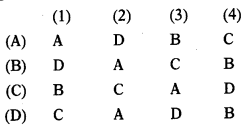(D)

Question 20.
A firm’s equity multiplier is an indication of its position.
(A) Liquidity
(B) Debt
(C) Asset utilization
(D) Inventory
(C) Asset utilization

Question 21.
Which of the following would NOT improve the current ratio
(A) Borrow short term to finance additional fixed assets.
(B) Issue long-term debt to buy inventory
(C) Sell common stock to reduce current liabilities
(D) Sell fixed assets to reduce accounts payable
(A) Borrow short term to finance additional fixed assets.

Question 22.
The gross profit margin is unchanged, but the net profit margin declined over the same period. This could have happened if
(A) Cost of goods sold increased relative to sales.
(B) Sales increased relative to expenses.
(C) The Indian government increased the tax rate.
(D) Dividends were decreased.
(C) The Indian government increased the tax rate.

Question 23.
P Ltd. has a debt-to-equity ratio of 1.6 compared with the industry average of 1.4. This means that the company
(A) Will not experience any difficulty with its creditors.
(B) Has less liquidity than other firms in the industry.
(C) Will be viewed as having high credit worthiness.
(D) Has greater than average financial risk when compared to other firms in its industry.
(D) Has greater than average financial risk when compared to other firms in its industry.

Question 24.
All of the following statements are true regarding ratios that measure a company’s ability to sell inventory and collect receivables except
(A) An increased accounts receivable turnover ratio indicates an increased ability to collect cash from credit customers.
(B) The formula for the inventory turn-over ratio is sales / average inventory.
(C) An increased inventory turnover ratio indicates a company is selling merchandise more quickly than in previous accounting periods; in general, this translates into more merchandise being sold and higher profitability.
(D) A decreased days sales in receivables ratio indicates the company is collecting cash from credit customers more quickly. In general, this results in greater cash inflows.
(B) The formula for the inventory turn-over ratio is sales / average inventory.

Question 25.
Which of the following statements (in general) is correct
(A) A low receivables turnover is desirable.
(B) The lower the total debt-to-equity ratio, the lower the financial risk for a firm.
(C) An increase in net profit margin with no change in sales or assets means a poor ROI.
(D) The higher the tax rate for a firm, the lower the interest coverage ratio.
(B) The lower the total debt-to-equity ratio, the lower the financial risk for a firm.

Question 26.
An examination of the sources and uses of funds statement is part of:
(A) A forecasting technique.
(B) A funds flow analysis.
(C) A ratio analysis
(D) Calculations for preparing the balance sheet.
(B) A funds flow analysis.

Question 27.
The DuPont Approach breaks down the earning power on shareholders’ book value (ROE) as follows: ROE =
(A) Net profit margin × Total asset turn-over × Equity multiplier
(B) Total asset turnover × Gross profit margin × Debt ratio
(C) Total asset turnover × Net profit margin
(D) Total asset turnover × Gross profit margin × Equity multiplier
(D) Total asset turnover × Gross profit margin × Equity multiplier

Question 28.
Which group of ratios measures a firm’s ability to meet short-term obligations
(A) Liquidity ratios
(B) Debt ratios
(C) Coverage ratios
(D) Profitability ratios
(A) Liquidity ratios

Question 29.
Which group of ratios relates the financial charges of a firm to its ability to service them
(A) Liquidity ratios
(B) Debt ratios
(C) Coverage ratios
(D) Profitability ratios
(C) Coverage ratios

Question 30.
All of the following statements are true regarding ratios that analyze a stock investment except
(A) In general, an increased P/E ratio indicates increased investor confidence in the future of the company.
(B) Shareholders who invest primarily to receive dividends pay special attention to the dividend yield ratio.
(C) Many experts argue that book value is the most useful ratio for investment analysis.
(D) Two ways for shareholders to earn a return on a share investment are receiving dividends and selling the stock investment at again.
(C) Many experts argue that book value is the most useful ratio for investment analysis.

Question 31.
Which group of ratios relate profits to sales and investment
(A) Liquidity ratios
(B) Debt ratios
(C) Coverage ratios
(D) Profitability ratios
(D) Profitability ratios

Question 32.
In Ratio Analysis, the term Capital Employed refers to:
(A) Equity Share Capital
(B) Net worth
(C) Shareholders Funds
(D) None of the above
(D) None of the above

Question 33.
Dividend Payout Ratio is:
(A) PAT/Capital
(B) DPS H-EPS
(C) Pref. Dividend 4- PAT
(D) Pref. Dividend 4- Equity Dividend
(B) DPS H-EPS

Question 34.
DuPont Analysis deals with:
(A) Analysis of Current Assets
(B) Analysis of Profit
(C) Capital Budgeting
(D) Analysis of Fixed Assets
(B) Analysis of Profit

Question 35.
In Net Profit Ratio, the denominator is:
(A) Net Purchases
(B) Net Sales
(C) Credit Sales
(D) Cost of goods sold
(B) Net Sales

Question 36.
Return on Investment may be improved by:
(A) Increasing Turnover
(B) Reducing Expenses
(C) Increasing Capital Utilization
(D) All of the above
(D) All of the above

Question 37.
Which group of ratios shows the extent to which the firm is financed with debt
(A) Liquidity ratios
(B) Debt ratios
(C) Coverage ratios
(D) Profitability ratios
(B) Debt ratios

Question 38.
Debt-Equity Ratio help to study
(A) Solvency
(B) Liquidity
(C) Profitability
(D) Turnover
(A) Solvency

Question 39.
Which of the following is considered a profitability measure
(A) Days sales in inventory
(B) Fixed asset turnover
(C) Price-earnings ratio
(D) Return on Assets
(D) Return on Assets

Question 40.
If the trend of the current ratio is increasing, while the trend of the acid-test ratio is decreasing over a period of time, this could be a warning that the firm is:
(A) Depleting its inventories
(B) Having trouble collecting its receivables
(C) Purchasing too much treasury stock
(D) Carrying excess inventories
(D) Carrying excess inventories

Question 41.
Return on Investment is computed as:
(A) Net income divided by average total assets
(B) Net income divided by average total owners’ equity
(C) Net income divided by total sales
(D) Sales divided by average total owners’ equity
(A) Net income divided by average total assets

Question 42.
Which of the following is not a category of financial statement ratios
(A) Financial leverage
(B) Liquidity
(C) Profitability
(D) Reliability
(D) Reliability

Question 43.
The dividend payout ratio describes:….
(A) The proportion of earnings paid as dividends
(B) The relationship of dividends per share to market price per share
(C) The percentage change in dividends this year compared to last year
(D) Dividends as a percentage of the price/earnings ratio
(A) The proportion of earnings paid as dividends

Question 44.
What type of ratios measure the liquidity of specific assets and the efficiency of managing assets
(A) Profitability Ratios
(B) Liquidity Ratios
(C) Leverage Ratios
(D) Activity Ratios
(D) Activity Ratios

Question 45.
Which of the following statements is false
(A) Financial ratios can serve as screening devices.
(B) No rules of thumb apply to the interpretation of financial ratios.
(C) Financial ratios are predictive.
(D) Financial ratios can indicate areas of potential strength and weakness.
(C) Financial ratios are predictive.

Question 46.
Which of the following ratios would be useful in assessing short-term liquidity
(A) Current ratio, quick ratio, cash-flow liquidity ratio.
(B) Average collection period, debt ratio, return on assets
(C) Quick ratio, accounts receivable turnover, return on assets.
(D) Current ratio, inventory turnover, fixed asset turnover
(A) Current ratio, quick ratio, cash-flow liquidity ratio.

Question 47.
What does a decreasing inventory turnover ratio usually indicate about a firm
(A) The firm is selling more inventory.
(B) The firm is managing its inventory well.
(C) The firm is inefficient in the management of inventory.
(D) Both (A) and (B)
(C) The firm is inefficient in the management of inventory.

Question 48.
What relationship exists between the average collection period and accounts receivable turnover
(A) As average collection period increases (decreases) the accounts receivable turnover decreases (increases)
(B) There is a direct and proportional relationship
(C) Both ratios are expressed in number of days.
(D) Both ratios are expressed in number of times receivables are collected per year.
(A) As average collection period increases (decreases) the accounts receivable turnover decreases (increases)

Question 49.
Company J and Company K each recently reported the same EPS. Company J’s stock, however, trades at a higher price. Which of the following statements is most correct
(A) Company J must have a higher P/E ratio.
(B) Company J must have a higher market to book ratio.
(C) Company J must be riskier.
(D) All of the statements above are correct.
(A) Company J must have a higher P/E ratio.

Question 50.
Which of the following statements is most correct ?
(A) If a company increases its current liabilities by ₹ 1,000 and simultaneously increases its inventories by ₹ 1,000, its current ratio must rise.
(B) If a company increases its current liabilities by ₹ 1,000 and simultaneously increases its inventories by ₹ 1,000, its quick ratio must fall.
(C) A company’s quick ratio may never exceed its current ratio.
(D) (B) & (C) is correct.
(D) (B) & (C) is correct.

Question 51.
Which of the following statements is most correct ?
(A) If two companies have the same return on equity, they should have the same stock price.
(B) If Company A has a higher profit margin and higher total assets turnover relative to Company B, then Company A must have a higher return on assets.
(C) If Company A and Company B have the same debt ratio, they must have the same times interest earned ratio.
(D) None of the above is correct.
(B) If Company A has a higher profit margin and higher total assets turnover relative to Company B, then Company A must have a higher return on assets.

Question 52.
Which of the following ratios is(are) useful in assessing a company’s ability to meet current maturing or short-term obligations ?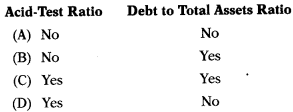(D)

Question 53.
Which of the following ratios should be used in evaluating the effectiveness with which the company uses its assets ?(D)

Question 54.
Activity ratios are measured with the help of …………
(A) Fixed assets
(B) Sales
(C) Profit
(D) Expenses
(B) Sales

Question 55.
Which of the following is not activity ratio
(A) Sales to inventory
(B) Net profit to sales
(C) Sales to debtors
(D) Assets turnover
(B) Net profit to sales

Question 56.
Debt equity ratio is …………..
(A) Profitability ratio
(B) Solvency Ratio
(C) Activity Ratio
(D) Operating Ratio
(B) Solvency Ratio

Question 57.
In capital gearing ratio nominator is
(A) Variable income securities
(B) Fixed income securities
(C) Both (A) & (B)
(D) Either (A) or (B)
(B) Fixed income securities

Question 58.
Issue of shares at cash will ……….. current ratio.
(A) Reduce
(B) Improve
(C) Not change
(D) Can’t say
(B) Improve

Question 59.
Purchase of stock for cash will ……….. current ratio.
(A) Reduce
(B) Improve
(C) Not change
(D) Can’t say
(C) Not change

Question 60.
Which of the following is market test ratio ?
(A) Basic Defense Interval
(B) Debt Service Coverage
(C) Dividend Yield Ratio
(D) All of the above
(C) Dividend Yield Ratio

Question 61.
Proprietary Ratio assess
(A) Long Term Solvency
(B) Short Term Solvency
(C) Profitability
(D) Activity
(A) Long Term Solvency

Question 62.
Proprietary Funds = …………..
(A) Equity Share Capital + Reserves – Fictitious Assets + Current assets
(B) Equity Share Capital + Preference Share Capital + Fixed Assets – Fictitious Assets
(C) Equity Share Capital + Preference Share Capital + Reserves – Fictitious Assets
(D) All of the above are correct
(C) Equity Share Capital + Preference Share Capital + Reserves – Fictitious Assets

Question 63.
Interest Cover is also known as………
(A) Debt Equity Ratio
(B) Debt Service-Ratio
(C) Debt Ratio
(D) Interest to debt ratio
(B) Debt Service-Ratio

Question 64.
While calculating dividend cover for preference shares numerator should be taken as ……….
(A) EBIT
(B) Profit available for equity shareholder
(C) PAT
(D) PAT + Depreciation
(C) PAT

Question 65.
……… ratios are used to measure how effectively the firm employs its resources.
(A) Turnover
(B) Profitability
(C) Market test
(D) Short term solvency
(A) Turnover

Question 66.
Which of the following statement is correct ?
(A) The higher the debtors turnover ratio betters the position.
(B) Lower the velocity betters the position.
(C) Both (A) & (B)
(D) None of the above
(C) Both (A) & (B)

Question 67.
The ……… reflects the market’s confidence in the company’s equity.
(A) P/E ratio
(B) Net profit ratio
(C) Cash profit ratio
(D) Total assets turnover ratio
(A) P/E ratio

Question 68.
…. is a good measure of the dividend policy of the company.
(A) Dividend Payout Ratio
(B) Price Earnings Ratio
(C) Earnings Per Share
(D) None of the above
(A) Dividend Payout Ratio

Question 69.
Explain the important ratio that would be used in following situation:
A bank is approached by a company for a loan of ₹ 50 lakh for working capital purposes.
(A) Capital Structure/Leverage Ratios
(B) Profitability Ratios
(C) Liquidity Ratios
(D) Activity Ratios
(C) Liquidity Ratios

Question 70.
A Ltd. financial statement shows the following data:
Opening stock ₹ 1,75,000, Total purchase ₹ 10,75,000 including cash purchase ₹ 1,75,000, total sales ₹ 15,00,000 out of which 20% are on cash basis. Closing stock is ₹ 1,50,000. Stock turnover ratio = ?
(A) 7.67
(B) 6.77
(C) 7.76
(D) 7.66
(B) 6.77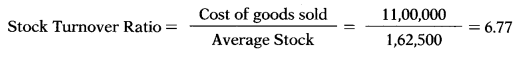Question 71.
A Ltd. financial statement shows the following data:
Equity ₹ 5,67,500, Reserve & surplus ₹ 3,87,850, total debt ₹ 5,88,778 out of which ₹ 2,88,778 Eire long term debt, fixed assets are ₹ 11,44,128.Current Ratio = ?
(A) 2.48
(B) 1.92
(C) 3.68
(D) 1.33
(D) 1.33
Current Liability = 5,88,778 – 2,88,778 = 3,00,000.
Current Assets = 5,67,500 + 3,87,850 + 5,88,778 – 11,44,128 = 4,00,000.Question 72.
Company’s EBIT is ₹ 2,99,000 which includes miscellaneous income ₹ 9,000. Its capital employed is ₹ 11,00,000. ROI = ?
(A) 27.18%
(B) 26.36%
(C) 26.18%
(D) 27.36%
(B) 26.36%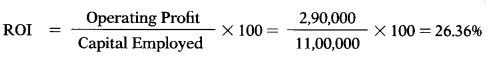Question 73.
KJ Ltd. PAT is ₹ 1,06,000. Its equity share capital is ₹ 3,50,000 of ₹ 10 each. The market price is ₹ 45.
P/E Ratio = ?
(A) 12.87
(B) 15.84
(C) 14.85
(D) 150
(C) 14.85

Question 74.
EBIT of NS Ltd. is ₹ 2,99,000 which includes miscellaneous income ₹ 9,000. Its capital structure consists of following long term debts: 12% Long-term loan ₹ 1,00,000 14% Debentures ₹ 2,50,000.
Interest Cover = ?
(A) 6.33
(B) 3.36
(C) 3.66
(D) 6.36
(D) 6.36

Question 75.
Current assets of Z Ltd. are ₹ 3,70,000 which includes stock ₹ 1,00,000 and prepstid expense ₹ 70,000. Its current liability are ₹ 1,60,000 which includes provision for tax ₹ 60,000. Liquid Ratio = ?
(A) 1.25
(B) 1.52
(C) 1.22
(D) 0.95
(A) 1.25Question 76.
KT Ltd. opening stock was ₹ 2,50,000 and closing stock was ₹ 3,75,000. Sales during the year was ₹ 13,00,000 and gross profit ratio was ₹ 25% on sales. Average account payable are ₹ 80,000. Creditors Turnover Ratio = ?
(A) 13.44
(B) 14.33
(C) 13.33
(D) 14.44
(A) 13.44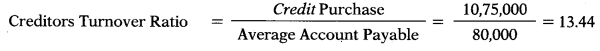Question 77.
Total sales of OLX Ltd. are ₹ 31,248 out of which 25% Eire cash stiles. Closing balance of debtors are ₹ 9,468. Debtors velocity = ?
(A) 4.2 months
(B) 157 days
(C) 148 days
(D) 4.43 months
(C) 148 days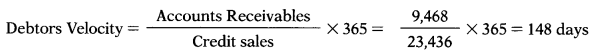Question 78.
Profit after tax of GB Ltd. was ₹ 3,40,000. Total assets are ₹ 5,94,70,000 out of which 3,57,00,000 was finance from loan funds.
Return on equity = ?
(A) 1.34%
(B) 0.57%
(C) 1.43%
(D) 1.57%
Use the following information to answer next 4 questions.
MNP Ltd. has made plans for the next. The company will employ total assets of ₹ 25,00,000; 30% of assets being financed by debt at an interest cost of 9% p.a. The direct costs for the year are estimated at ₹ 15,00,000 and all other operating expenses are estimated at ₹ 2,40,000. The sales revenue Eire estimated at ₹ 22,50,000. Tax rate is assumed to be 40%.
(C) 1.43%Question 79.
Net profit margin ratio = ?
(A) 10.62%
(B) 11.8%
(C) 15.17%
(D) 11.86%
(B) 11.8%Question 80.
Return on Assets = ?
(A) 12.46%
(B) 15.17%
(C) 11.86%
(D) 10.62%
(D) 10.62%Question 81.
Asset Turnover Ratio = ?
(A) 0.9
(B) 1.1
(C) 1.9
(D) 1.33
(A) 0.9Question 82.
Return on equity = ?
(A) 12.46%
(B) 15.17%
(C) 11.86%
(D) 12.46%
(B) 15.17%Question 83.
N Ltd. gives the following information:
Current Ratio 2.8
Total assets ₹ 60,00,000
Fixed assets ₹ 32,00,000
Current liabilities = ?
(A) ₹ 28,00,000
(B) ₹ 10,00,000
(C) ₹ 18,00,000
(D) ₹ 12,00,000
(B) ₹ 10,00,000
Total assets – Fixed assets = Current assets
60,00,000 – 32,00,000 = 28,00,0002.8x = 28,00,000
x = Current Liabilities = 10,00,000

Question 84.
N Ltd. gives the following information:
Liquid ratio — ₹ 1.6
Current Assets — ₹ 28,00,000
Current Liabilities — ₹ 10,00,000
Stock = ?
(A) ₹ 28,00,000
(B) ₹ 10,00,000
(C) ₹ 18,00,000
(D) ₹ 12,00,000
(D) ₹ 12,00,000Question 85.
S Ltd. gives the following information:
Net working capital — ₹ 2,80,000
Current ratio — 2.4
Liquid ratio — 1.6
Current Assets = ?
(A) ₹ 2,00,000
(B) ₹ 2,80,000
(C) ₹ 4,80,000
(D) ₹ 3,60,000
(C) ₹ 4,80,00024y = x
CA – CL = WC
x – y = 2,80,000
2.4y – y= 2,80,000
1.4y = 2,80,000
y = Current Liabilities = 2,00,000
x = Current Assets 2,00,000 × 2.4 4,80,000

Question 86.
Z Ltd. gives the following information: Capital block to current assets = 3:2 Current Assets = ₹ 8,00,000 Debenture/share capital =1:2
Net profit = 10% of turnover Reserve = 2.5% of turnover Total Sales = ₹ 24,00,000 Share capital = ?
(A) ₹ 3,00,000
(B) ₹ 6,60,500
(C) ₹ 14,00,000
(D) ₹ 6,00,000
(D) ₹ 6,00,000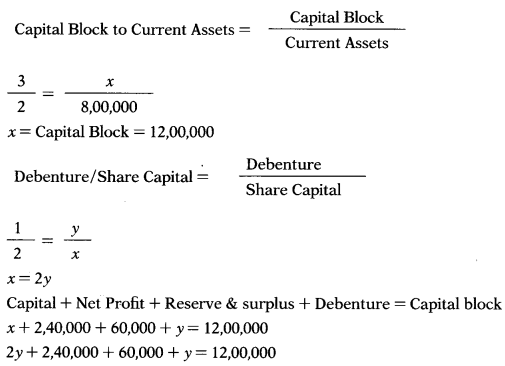3y = 900,000
y = Debenture 3,00,000
x = Share capital 6,00,000

Question 87.
N Ltd. gives the following information: Fixed assets = ₹ 10,50,000
Fixed assets turnover ratio (on cost of sales) = 2
GP rate on sales = 25%
Sales = ?
(A) ₹ 21,00,000
(B) ₹ 25,00,000
(C) ₹ 26,00,000
(D) ₹ 28,00,000
(D) ₹ 28,00,000x = Cost of sales = 21,00,000
Gross profit is 25% on sales that means 1/3rd or 33.33% on cost.
Gross profit = 21,00,000 × 1/3 = 7,00,000
Cost of sales + Gross profit = Sales
21,00,000 + 7,00,000 = 28,00,000

Question 88.
NS Ltd. gives the following information: Current Ratio = 2.4
Quick Ratio =1.0
Stock = ₹ 5,60,000 Current Assets = ?
(A) ₹ 9,60,000
(B) ₹ 6,90,000
(C) ₹ 4,00,000
(D) ₹ 4,60,000
(A) ₹ 9,60,000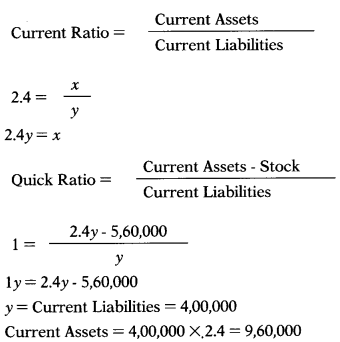Question 89.
SZ Ltd. gives the following information: Fixed Assets = 10,50,000
Current Assets = 9,60,000
Current Liabilities = 4,00,000
Debenture = 4,00,000
Reserve to capital = 0.21
Reserve = ?
(A) ₹ 10,00,000
(B) ₹ 12,10,000
(C) ₹ 2,10,000
(D) ₹ 1,10,000
(C) ₹ 2,10,000
Fixed assets + Current assets – current liabilities – debenture Net worth
10,50,000 + 9,60,000 – 4,00,000 – 4,00,000 = 12,10,0000.21y = x
Capital + Reserve = Net worth
y + x = 12,10,000
y + 0.21y= 12,10,000
1.21y = 12,10,000
y = Capital = 10,00,000
x = Reserve = 10,00,000 × 0.21 = 2,10,000

Question 90.
Gross profit ratio = 20%.
Sales = ₹ 25,00,000;
Stock velocity = 6
Gross profit for the year ended amounts to ₹ 5,00,000. Stock of the year is ₹ 20,000 more than what it was at the beginning of the year. Closing stock = ?
(A) ₹ 10,00,000
(B) ₹ 9,90,000
(C) ₹ 10,10,000
(D) ₹ 10,20,000
(C) ₹ 10,10,000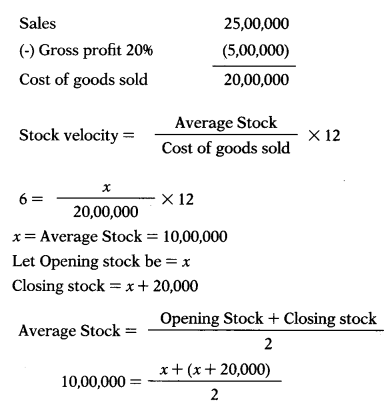20,00.000 2x + 20,000
19,80,000 = 2x
x = Opening stock = 9,90,000
Closing stock = 9,90,000 + 20,000 = 10,10,000

Question 91.
Debtors velocity = 3 months Sales = 25,00,000
Bills receivable & Bills payable were ₹ 60,000 and 36,667 respectively. Sundry debtors = ?
(A) ₹ 6,25,000
(B) ₹ 5,25,000
(C) ₹ 5,65,000
(D) ₹ 6,65,000
(C) ₹ 5,65,000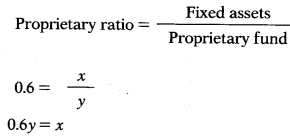Question 92.
Creditors velocity = 2 months.
Cost of goods sold = ₹ 20,00,000
Opening stock = ₹ 9,90,000
Closing stock = ₹ 10,10,000
Bills receivable & Bills payable were ₹ 60,000 and 36,667 respectively.
Creditors = ?
(A) ₹ 3,36,667
(B) ₹ 3,66,333
(C) ₹ 3,30,367
(D) ₹ 3,00,000
(D) ₹ 3,00,000x = Accounts payable = 3,36,667
Creditors + Bills payable = Account payable
x + 36,667 = 3,36,667
x = Creditors = ₹ 3,00,000

Question 93.
Capital gearing ratio = 0.625
Long term debts = ₹ 5,00,000
Reserve to capital = 0.6
Reserve = ?
(A) 8,00,000
(B) 5,00,000
(C) 3,00,000
(D) 4,00,000
(C) 3,00,000Capital + Reserve = Net worth
y+ x 8,00,000
y + 0.6y = 8,00000
1.6y = 8,00,000
y = Capital = 5,00,000
x = Reserve = 5,00,000 × 0.6 = 3,00,000

Question 94.
Proprietary Ratio = 0.6 [Fixed Assets/Proprietary Fund]
Current Assets = ₹ 2,00,000
Current Liabilities = ₹ 80,000
Fixed Assets = ₹
(A) 3,00,000
(B) 1,60,000
(C) 1,80,000
(D) 2,40,000
Use the following information to answer next 5 question.
Gross profit 25% of sales Gross profit = 11,20,000 Shareholders equity = ₹ 20,000 Credit sales to total sales 80%
Total turnover to total assets = 4 times Cost of sales to inventory =10 times
Average collection period = 5 days, assume 365 days in year.
Current ratio =1.5 Sundry creditor = ₹ 60,000
(C) 1,80,000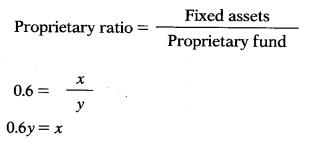Proprietary Fund + Current Liabilities = Fixed Assets + Current Assets y + 80,000 = x + 2,00,000
y + 80,000 = 0.6y + 2,00,000 0.4 y= 1,20,000
1,20,000
y = Proprietary fund = $$\frac{1,20,000}{0.4}$$= 3,00,000
x = Fixed assets = 3,00,000 × 0.6 = 1,80,000

Question 95.
Stock = ?
(A) 36,000
(B) 32,000
(C) 40,000
(D) 42,000
(A) 36,000
Calculation of sales & cost of goods sold:Sales = 4,80,000
Sales – Gross Profit = Cost of sales
4,80,000 – 1,20,000 = 3,60,000Calculation of current assets:
$$1.5=\frac{\text { Current Assets }}{60,000}$$
Current Assets = 90,000
Calculation of cash:
Cash + Sundry Debtors + Inventory Current Assets
Cash + 5,260 + 36,000 = 90,000
Cash = 48,740

Question 96.
Debtors = ?
(A) 5,260
(B) 5,620
(C) 6,520
(D) 2,650
(A) 5,260
Calculation of sales & cost of goods sold:Sales = 4,80,000
Sales – Gross Profit = Cost of sales
4,80,000 – 1,20,000 = 3,60,000Calculation of current assets:
$$1.5=\frac{\text { Current Assets }}{60,000}$$
Current Assets = 90,000
Calculation of cash:
Cash + Sundry Debtors + Inventory Current Assets
Cash + 5,260 + 36,000 = 90,000
Cash = 48,740

Question 97.
Current Assets = ?
(A) 1,20,000
(B) 1,10,000
(C) 1,00,000
(D) 90,000
(D) 90,000
Calculation of sales & cost of goods sold:Sales = 4,80,000
Sales – Gross Profit = Cost of sales
4,80,000 – 1,20,000 = 3,60,000Calculation of current assets:
$$1.5=\frac{\text { Current Assets }}{60,000}$$
Current Assets = 90,000
Calculation of cash:
Cash + Sundry Debtors + Inventory Current Assets
Cash + 5,260 + 36,000 = 90,000
Cash = 48,740

Question 98.
Cash = ?
(A) 48,470
(B) 48,740
(C) 44,870
(D) 47,470
(B) 48,740
Calculation of sales & cost of goods sold:Sales = 4,80,000
Sales – Gross Profit = Cost of sales
4,80,000 – 1,20,000 = 3,60,000Calculation of current assets:
$$1.5=\frac{\text { Current Assets }}{60,000}$$
Current Assets = 90,000
Calculation of cash:
Cash + Sundry Debtors + Inventory Current Assets
Cash + 5,260 + 36,000 = 90,000
Cash = 48,740

Question 99.
Total of Balance Sheet = ?
(A) 1,20,000
(B) 1,40,000
(C) 1,30,000
(D) 1,25,000
(A) 1,20,000
Calculation of sales & cost of goods sold:Sales = 4,80,000
Sales – Gross Profit = Cost of sales
4,80,000 – 1,20,000 = 3,60,000Calculation of current assets:
$$1.5=\frac{\text { Current Assets }}{60,000}$$
Current Assets = 90,000
Calculation of cash:
Cash + Sundry Debtors + Inventory Current Assets
Cash + 5,260 + 36,000 = 90,000
Cash = 48,740

Question 100.
When the current ratio is 2:5, and the amount of current liabilities is ₹ 25,000, what is the amount of current assets?
(A) ₹ 62,500
(B) ₹ 12,500
(C) ₹ 10,000
(D) None of these
(C) ₹ 10,000

Question 101.
When quick ratio is 1.5:1 and the amount of quick assets ₹ 30,000, what is the amount of quick liabilities?
(A) ₹ 20,000
(B) ₹ 50,000
(C) ₹ 45,000
(D) ₹ 30,000
(A) ₹ 20,000

Question 102.
When opening stock is ₹ 50,000, closing stock ₹ 60,000, and cost of goods sold ₹ 2,20,000, the stock turnover ratio is ………..
(A) 2 times
(B) 3 times
(C) 4 times
(D) 5 times
(C) 4 times

Question 103.
When net sales for the year are ₹ 2,50,000 and debtors ₹ 50,000, the average collection period is:
(A) 60 days
(B) 45 days
(C) 42 days
(D) 73 days
(D) 73 days

Question 104.
Given net profit ₹ 150,000, preference dividend ₹ 25,000, taxes ₹ 10,000 and number of equity shares 1,00,000. What is the Earning per Share (EPS)?
(A) ₹ 1.50
(B) ₹ 1.25
(C) ₹ 1.15
(D) None of these
(C) ₹ 1.15

Question 105.
When net profit is ₹ 2,25,000, taxes ₹ 25,000 and net worth ₹ 10,00,000 what is the rate of return on shareholders’ equity
(A) 22.5%
(B) 2096
(C) 2596
(D) Cannot be calculated
(B) 2096

Question 106.
Accounting information given by a company:
Total assets turnover 3 times
Net Profit margin 1096
Total assets ₹ 1,00,000
The net profit is:
(A) ₹ 10,000
(B) ₹ 15,000
(C) ₹ 25,000
(D) ₹ 30,000
(D) ₹ 30,000

Question 107.
Balance Sheet of a company indicates that its current ratio is 1.5. Company’s net working capital is ₹ 1 crore. The Current Assets would amount to
(A) ₹ 3 Crore
(B) ₹ 1.5 Crore
(C) ₹ 4 Crore
(D) ₹ 2.5 Crore
(A) ₹ 3 Crore

Question 108.
Earnings after Interest and Tax is ₹ 20 Crore, interest is ₹ 4 Crore, Income Tax is ₹ 16 Crore. Interest Coverage Ratio would be
(A) 10.00
(B) 9.00
(C) 7.50
(D) 5.00
(A) 10.00

Question 109.
For the financial year ended as on March 31,2013 the figures extracted from the balance sheet of X Ltd. as under:
Opening Stock ₹ 29,000;
Purchases ₹ 2,42,000;
Sales ₹ 3,20,000;
Gross Profit 25% of Sales.
Stock Turnover Ratio will be :
(A) 8 times
(B) 6 times
(C) 9 times
(D) 10 times
(A) 8 times

Question 110.
If credit sales for the year is ₹ 5,40,000 and Debtors at the end of year is ₹ 90,000 the Average Collection Period will be ………….. ?
(A) 30 days
(B) 61 days
(C) 90 days
(D) 120 days
(B) 61 days

Question 111.
Given the following information: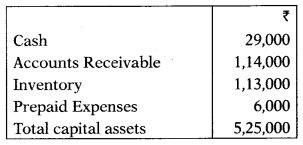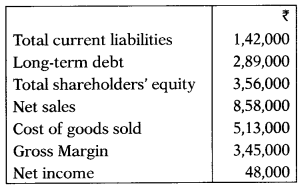The acid test ratio = ?
(A) 75%
(B) 1.85 times
(C) 1.01 times
(D) 55%
(A) 75%

Question 112.
Given the following information: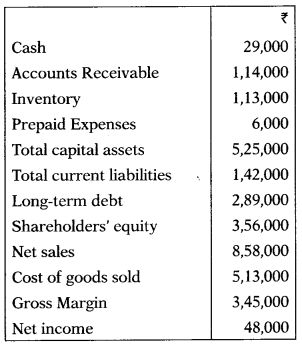(A) 8.62
(B) 5.56
(C) 7.52
(D) 4.54
(D) 4.54

Question 113.
Determine a firm’s total asset turnover if its net profit margin is 5%, total assets is ₹ 80,00,000 and ROI is 8%.
(A) 1.60
(B) 2.05
(C) 2.50
(D) 4.00
(A) 1.60

Question 114.
X Ltd. has an 8% return on total assets of ₹ 3,00,000 and a net profit margin of 5%. What are its sales?
(A) ₹ 37,50,000
(B) ₹ 4,80,000
(C) ₹ 3,00,000
(D) ₹ 15,00,000
(B) ₹ 4,80,000

Question 115.
K Ltd. had sales last year of ₹ 26,50,000, including cash sales of ₹ 2,50,000. If its average collection period was 36 days, its ending accounts receivable balance is closest to (Assume a 365 day year.)
(A) 2,40,000
(B) 2,36,712
(C) 2,63,127
(D) 2,40,721
(B) 2,36,712

Question 116.
K Ltd. has debt-to-total assets 0.4. What is its debt-to-equity ratio?
(A) 0.2
(B) 0.6
(C) 0.667
(D) 0.333
(C) 0.667

Question 117.
Firm A has a Return on Equity equal to 24%, while firm B has an ROE of 15% during the same year. Both firms have a total debt ratio equal to 0.8. Firm A has an asset turnover ratio of 0.9, while firm B has an asset turnover ratio equal to 0.4. From this we know that
(A) Firm A has a higher profit margin than firm B
(B) Firm B has a higher profit margin than firm A
(C) Firm A and B have the same profit margin
(D) Firm A has a higher equity multiplier than firm B
Use the following information to answer next 5 questions:
XL Ltd. gives the following data:(B) Firm B has a higher profit margin than firm A

Question 118.
Current Ratio = ?
(A) 2.1
(B) 1.1
(C) 0.1
(D) 0.9
(B) 1.1

Question 119.
Acid Test Ratio = ?
(A) 0.9
(B) 1.1
(C) 0.75
(D) 0.87
(C) 0.75

Question 120.
Debtors Velocity = ?
(A) 9 days
(B) 24 days
(C) 4 days
(D) 15 days
(D) 15 days

Question 121.
Inventory Turnover Ratio =
(A) 7
(B) 14
(C) 18
(D) 9
(B) 14

Question 122.
(A) 35 days
(B) 5 days
(C) 20 days
(D) (11) days
Use the following information to answer next 4 questions:Gross profit for the year amounts to ₹ 10,00,000. Closing stock of the year is ₹ 40,000 above the opening stock. Bills receivable amount to ₹ 1,20,000 and Bills payable to ₹ 73,333.
(D) (11) days

Question 123.
Sales = ?
(A) 52,50,000
(B) 47,50,000
(C) 40,00,000
(D) 50,00,000
(D) 50,00,000

Question 124.
Sundry debtors = ?
(A) 12,50,000
(B) 11,50,000
(C) 11,30,000
(D) 12,30,000
(C) 11,30,000

Question 125.
Closing stock = ?
(A) 19,80,000
(B) 20,20,000
(C) 19,60,000
(D) 20,40,000
(B) 20,20,000

Question 126.
Sundry creditors ?
(A) 6,73,333
(B) 6,66,667
(C) 6,00,000
(D) 5,73,667
(C) 6,00,000

Question 127.
From the information given below calculate the amount of fixed assets. Fixed assets to proprietors fund = 0.75 Net Working Capital = ₹ 6,00,000
(A) 17,50,000
(B) 18,00,000
(C) 17,00,000
(D) 18,50,000
(B) 18,00,000

Question 128.
If a firm has ₹ 100 in inventories, a current ratio equal to 1.2, and a quick ratio equal to 1.1, what is the firm’s Net Working Capital?
(A) ₹ 10
(B) ₹ 100
(C) ₹ 200
(D) ₹ 1,200
(C) ₹ 200

Question 129.
Given the following information, calculate the company’s long-term debt.
Current assets: — ₹ 1,25,000
Current liabilities: — ₹ 85,000
Net fixed assets: — ₹ 2,50,000
Total equity: — ₹ 2,00,000
(A) ₹ 3,75,000
(B) ₹ 50,000
(C) ₹ 2,85,000
(D) ₹ 90,000
(D) ₹ 90,000

Question 130.
G Ltd. has total current liabilities of ₹ 2,000 and an inventory of ₹ 1,000. If its current ratio is 2.5, then what is its quick ratio.
(A) 2.0
(B) 2.5
(C) 3.0
(D) 3.5
(A) 2.0

Question 131.
Dec 2014: Find the current liability from the following:
Current ratio – 2:5 Liquid ratio – 1:5 Prepaid expenses – Nil Stock – ₹ 4,000
(A) ₹ 20,000
(B) ₹ 40,000
(C) ₹ 80,000
(D) ₹ 4,000
(A) ₹ 20,000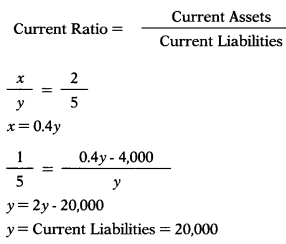Question 132.
Dec 2014: In an organization, profit after interest, tax and dividend on preference shares is ₹ 4,00,000. The number of equity shares is 40,000 and the dividend payout ratio is 40%. The dividend per share is —
(A) ₹ 4
(B) ₹ 25
(C) ₹ 10
(D) ₹ 6
(A) ₹ 4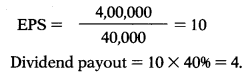Question 133.
Dec 2014: From the following information find the value of closing stock —
Stock velocity: 6 months Gross profit ratio: 25%
Gross profit for the year ended 31 st March 2014: ₹ 1,00,000
Closing stock for the period – ₹ 20,000 more than it was in the beginning of the year.
(A) ₹ 1,50,000
(B) ₹ 1,40,000
(C) ₹ 1,60,000
(D) ₹ 70,000
(C) ₹ 1,60,000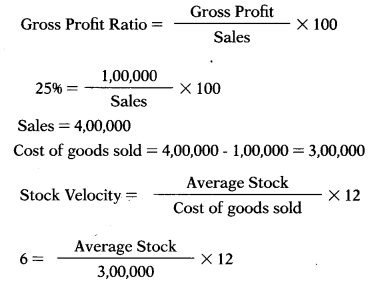Average Stock = 1,50,000
Let Opening stock be = x
Closing stock = x + 20,0003,00,000 = 2x + 20,000
2,80,000 = 2x
x = Opening stock = 1,40,000
Closing stock = 1,40,000 + 20,000
= 1,60,000

Question 134.
June 2015: The net profit of a company is ₹ 2,00,000, preference dividend ₹ 25,000 and taxes paid ₹ 15,000. Number of equity shares is 1,00,000. The earnings per share (EPS) is –
(A) ₹ 1.5
(B) ₹ 1.6
(C) ₹ 2
(D) ₹ 1.75
(B) ₹ 1.6Question 135.
June 2015: The current ratio of Brave Ltd. is 2:1, while quick ratio is 1.8:1. If the current liabilities are ₹ 40,000, the value of stock will be –
(A) ₹ 12,000
(B) ₹ 6,500
(C) ₹ 8,000
(D) ₹ 10,000
(C) ₹ 8,000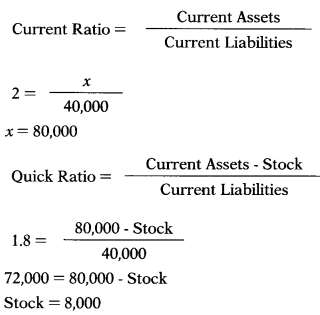Question 136.
June 2015: In an organization, working capital is ₹ 1,00,000 and current ratio 3:1. The value of current assets is –
(A) ₹ 1,50,000
(B) ₹ 1,00,000
(C) ₹ 50,000
(D) ₹ 15,000
(A) ₹ 1,50,000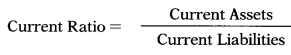Question 137.
June 2015: Working capital ratio is also known as –
(A) Quick ratio
(B) Debt-Equity ratio
(C) Current ratio
(D) Liquid ratio
(C) Current ratio

Question 138.
June 2015: Credit sales of Jump Ltd. for the year is ₹ 12,00,000 and debtors at the end of year ₹ 2,40,000. Assuming 360 days in a year, average collection period will be –
(A) 60 Days
(B) 72 Days
(C) 180 Days
(D) 80 Days
(B) 72 DaysQuestion 139.
June 2015: For the financial year ended 31 st March 2015, the figures extracted from the balance sheet of Excel Ltd. are as under:
Opening stock ₹ 29,000
Closing stock ₹ 31,000
Purchases ₹ 2,42,000
The stock turnover ratio will be –
(A) 12 Times
(B) 15 Times
(C) 9 Times
(D) 8 Times
(D) 8 Times
Opening Stock + Purchase – Closing Stock = Cost of goods sold
29.000 + 2,42,000 – 31,000 = 2,40,000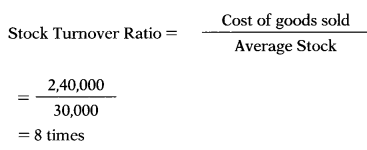Question 140.
June 2015: Which of the following is a method used in analyzing financial statements –
(A) Variance analysis
(B) Trend analysis
(C) Break-even analysis
(D) Budget analysis
(B) Trend analysis

Question 141.
June 2015: In an organization, current ratio is 2.5, liquid ratio 1.5, prepaid expenses nil and stock ₹ 4,000. The amount of current liabilities is –
(A) ₹ 20,000
(B) ₹ 40,000
(C) ₹ 80,000
(D) ₹ 4,000
(D) ₹ 4,000

Question 142.
Dec 2015: ……. are necessary for the study of trends and direction of movements in the financial position and operating results of a concern.
(A) Trend ratios
(B) Cash flow statements
(C) Common size statements
(D) Comparative statements
(A) Trend ratios

Question 143.
Dec 2015: Current ratio is 2.5 and liquid ratio is 1.5. Working capital is ₹ 75,000. Value of the stock held will be —
(A) ₹ 60,000
(B) ₹ 1,00,000
(C) ₹ 50,000
(D) None of the above
(C) ₹ 50,000Question 144.
Dec 2015: Determine a firm’s total assets turnover, if its net profits margin is 8%, total assets are ₹ 8,00,000 and the return on investment is 14% —
(A) 2.05
(B) 4.00
(C) 1.75
(D) 2.00
(C) 1.75
14%/8% =1.75

Question 145.
Dec 2015: Net income of a company after payment of preference dividend was ₹ 63 lakh. The number of equity shares was 1,40,000. The P/E ratio of the company was 8.50 times. Earnings per share and market value per share would be —
(A) ₹ 45 & ₹ 382.50 respectively
(B) ₹ 45 & ₹ 308.20 respectively
(C) ₹ 33.16 & ₹ 281.86 respectively
(D) ₹ 45 & ₹ 5.29 respectively
(A) ₹ 45 & ₹ 382.50 respectively
$$\mathrm{EPS}=\frac{63,00,000}{1,40,000}=45$$
Market Price = P/E Ratio × EPS
Market Price = 8.5 × 45
Market Price = 382.5

Question 146.
Dec 2015: In ratio analysis, ‘proforma analysis’ implies —
(A) Making a list of all the present ratios of the firm
(B) Comparison of liquidity ratios with other kind of ratios of the firm
(C) Comparison of the ratios of the firm relating to the performance of the firm
(D) Comparison of the firm’s past and current ratios with future ratios to ascertain the relative strengths and weaknesses in the past and future
(D) Comparison of the firm’s past and current ratios with future ratios to ascertain the relative strengths and weaknesses in the past and future

Question 147.
Dec 2015: Match the following:
List-I — List-II
P. Prepaid expenses — 1. Solvency ratio
Q. Sales ratio — 2. Net profit margin X Investment ratio
R. Return on investment — 3. Turnover ratio
S. 100 minus Proprietary — 4. Current asset ratio
Select the correct answer from the options given below —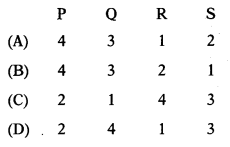(B)

Question 148.
Dec2015: Which of the following pairs is correctly matched —
(A) Administrative expenses + Selling and distribution expenses = Operating expenses
(B) (Gross profit 4- Net sales) × 100 = Net profit ratio
(C) Both (A) and (B) above
(D) None of the above
(D) None of the above

Question 149.
Dec 2015:
Statement-I:
If any fixed asset remains idle due to abnormal or unusual events, it should be included in capital employed.
Statement-II:
Idle machines and tools required for normal operation of plant would not be included in capital employed.
Select the correct answer from the following
(A) Both statements are correct
(B) Both statements are incorrect
(C) Statement-I is correct, but Statement-II is incorrect
(D) Statement-I is incorrect, but Statement-II is correct
(B) Both statements are incorrect

Question 150.
Dec 2015:
Assertion (A):
Accounting ratios reveal the financial position of a concern.
Reason (R):
Accounting ratios are not useful in assessing the operational efficiency. Select the correct answer from the following —
(A) Both A and R are true and R is the correct explanation of A
(B) Both A and R are true, but R is not the correct explanation of A
(C) A is true, but R is false
(D) A is false, but R is true
(C) A is true, but R is false

Question 151.
Dec 2015: Return on investment depends on two ratios —
(A) Net profit ratio and capital turnover ratio
(B) Gross profit ratio and net profit ratio
(C) Capital employed ratio and assets turnover ratio
(D) Earnings per share and net profit ratio
(A) Net profit ratio and capital turnover ratio

Question 152.
Which of the following pairs is not correctly matched —
(A) Dividend per equity share/Earnings per share = Payout ratio
(B) [Operating profit/Capital employed] × 100 = Return on capital employed
(C) [(Cost of goods sold + operating expenses)/ net sales] × 100 = Operating profit ratio
(D) None of the above
(D) None of the above

Question 153.
Dec 2015: Match the following:
List – I — List – II
P. Operating profit 1. Capital employed = …….. + Preference share capital
Q. Liquid liabilities — 2. ……….. = Gross profit – Operating expenses
R. Capital employed 3. Quick assets = Quick ratio × ………
S. Equity share capital — 4. Fixed assets ratio = fixed assets ÷
Select the correct ans were from the options given below —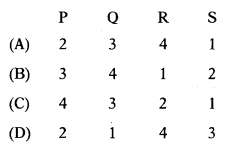(A)

Question 154.
Dec 2015: Which of the following pairs is correctly matched —
(A) Profitability ratios – Expenses ratios
(B) Activity ratios – Total assets turnover ratio
(C) Both (A) and (B) above
(D) None of the above
(B) Activity ratios – Total assets turnover ratio

Question 155.
Return on investment is also known as —
(A) DuPont chart
(B) Activity ratio
(C) P/V ratio
(D) Market test ratio
(A) DuPont chart

Question 156.
Dec 2015:
Assertion (A):
Higher the gross profit ratio, the better it is. Reason (R):
A low gross profit ratio indicates unfavourable trend in the form of reduction in selling prices.
Select the correct answer from the following
(A) Both A and R are true and R is the correct explanation of A
(B) Both A and R are true, but R is not the correct explanation of A
(C) A is true, but R is false
(D) A is false, but R is true
(A) Both A and R are true and R is the correct explanation of A

Question 157.
Dec 2015: Equity share capital: ₹ 30 lakh (30,000 shares of ₹ 100 each); 9% preference shares: ₹ 10 lakh; profit before tax: ₹ 24.46 lakh and tax rate 30%. Earnings per share will be —
(A) ₹ 54.07
(B) ₹ 81.53
(C) ₹ 78.53
(D) ₹ 57.07
(A) ₹ 54.07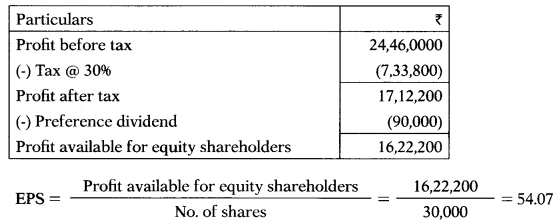Question 158.
Dec 2015: Financial statement of X Ltd. shows the following data —
Opening stock — 1,50,000
Total purchases (including cash — 10,50,000
purchases of — ₹ 1,75,000)
Closing stock — ₹ 1,20,000
Stock turnover ratio is —
(A) 6.70 times
(B) 8 times
(C) 7.2 times
(D) 9 times
(B) 8 timesQuestion 159.
June 2016: In financial analysis, ‘time series analysis’ refers to —
(A) Making a time series of various ratios to assess a firm’s profitability
(B) A graphical comparison of a firm’s sources of finance
(C) The comparison of financial ratios over a period of time to assess the direction of change and the financial performance of a firm
(D) A comparison of time values for various ratios of a firm
(C) The comparison of financial ratios over a period of time to assess the direction of change and the financial performance of a firm

Question 160.
June 2016: If average collection period is 15 days and average account receivables is ₹ 45,000, the total amount of credit sales will be (assume 360 days in a year) —
(A) ₹ 10,80,000
(B) ₹ 16,20,000
(C) ₹ 6,75,000
(D) ₹ 1,87,500
(A) ₹ 10,80,000

Question 161.
June 2016:
Cost of goods sold — 4,00,000
Admin. & office exp. — 35,000
Selling & distribution exp. — 45,000
Net credit sales — 4,75,000
Cash sales — 1,25,000
Operating profit ratio will be —
(A) 30%
(B) 35%
(C) 20%
(D) 25%
(C) 20%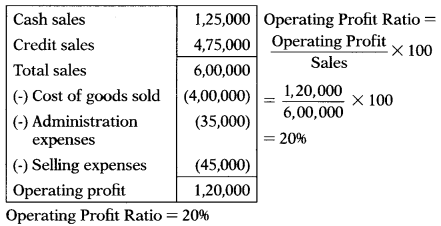Question 162.
June 2016: Current liabilities of a firm are ₹ 1,50,000. Its current ratio is 3:1 and liquid ratio is 1:1. The value of stock will be —
(A) ₹ 3,00,000
(B) ₹ 4,50,000
(C) ₹ 2,50,000
(D) ₹ 1,50,000
(A) ₹ 3,00,000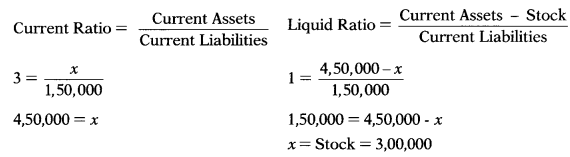Question 163.
June 2016:
Assertion (A):
A current ratio of 2:1 is considered satisfactory as a rule of thumb but it should not be followed blindly.
Reason (R):
The greatest weakness of current ratio is the possibility of window dressing and manipulation.
Select the correct answer from the options given below —
(A) Both A and R are true and R is the correct explanation of A
(B) Both A and R are true, but R is not the correct explanation of A
(C) A is true, but R is false
(D) A is false, but R is true
(A) Both A and R are true and R is the correct explanation of A

Question 164.
June 2016:
Stock turnover: 6 times
Total sales: ₹ 3,00,000
Gross profit ratio: 20%
Closing stock: ₹ 4,000 more than opening stock
The opening stock is —
(A) ₹ 36,000
(B) ₹ 38,000
(C) ₹ 40,000
(D) ₹ 42,000
(B) ₹ 38,000

Question 165.
June 2016:Which one of the following statements is correct —
(A) Lower debt equity ratio means lower financial risk
(B) Increase in net profit ratio means increase in sales
(C) A higher receivable turnover is not desirable
(D) Interest coverage ratio depends upon tax rate
(A) Lower debt equity ratio means lower financial risk

Question 166.
June 2016: Which of the following statement(s) is /are true:
(i) Common size balance sheet is vertical financial analysis
(ii) Financial analysis performed on behalf of shareholders is called internal analysis
(iii) Trend percentage may be used for both balance sheet and profit and loss account.
Select the correct answer from the options given below —
(A) (i) and (ii)
(B) (ii) and (iii)
(C) (i) and (iii)
(D) (ii) only
(C) (i) and (iii)

Question 167.
June 2016: From the following financial data, compute stock turnover ratio and stock velocity (assume 360 days in a year) —
Opening stock : — 58,000
Purchases : — 5,02,000
Return outwards : — 18,000
Sales : — 6,53,000
Return inwards : — 13,000
Gross profit :25% on sales
Choose the correct option —
(A) 8 times; 45.62 days
(B) 8 times; 45 days
(C) 10.67 times; 33.75 days
(D) 7.74 times; 46.51 days
(B) 8 times; 45 days
Net Purchases = 5,02,000 – 18,000 = 4,84,000
Net Sales 6,53,000 – 13,000 = 6,40,000
Cost of goods sold = 6,40,000 – 1,60,000 (25% profit on sales) = 4,80,000
Opening Stock + Purchases – Closing Stock = Cost of goods sold
58,000 + 4,84,000 – x = 4,80,0000
x = Closing Stock = 62,000Question 168.
June 2016:
% Preference share capital : — ₹ 3,00,000
Equity share capital : — ₹ 8,00,000
(₹ 10 per share)
Profit after 3096 tax : — ₹ 2,80,000
Market price of equity share : — ₹ 40
The earnings per share and the price
earnings ratio will be —
(A) ₹ 3.50 and 11.43
(B) ₹ 5 and 8
(C) ₹ 4.70 and 8.51
(D) ₹ 3.20 and 12.50
(D) ₹ 3.20 and 12.50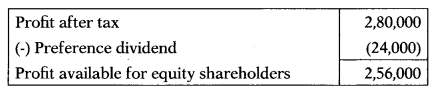$$\mathrm{EPS}=\frac{2,56,000}{80,000}=3.2$$
$$\mathrm{P} / \mathrm{E} \text { Ratio }=\frac{40}{3.2}=12.5$$

Question 169.
Dec2016: Return on investment (ROI) is calculated to measure —
(B) Earning power of net assets of business
(C) Short-term liquidity position of business
(D) Goods sold and inventory level of business
(B) Earning power of net assets of business

Question 170.
Dec 2016: Interest coverage ratio is obtained by dividing EBIT by —
(A) Interest
(B) Tax
(C) Income
(D) Sales
(A) Interest

Question 171.
Dec 2016: If price-earnings ratio is 0.05 and earnings per share is ₹ 8, the market price of share will be —
(A) ₹ 120
(B) ₹ 100
(C) ₹ 160
(D) ₹ 0.40
(D) ₹ 0.40
Market price = EPS × P/E Ratio = 8 × 0.05 = 0.4

Question 172.
Dec 2016: Sun Ltd. has furnished the following relevant data of financial statements as on 31st March, 2016:
Equity share capital (1,00,000 equity shares of ₹ 10 each) — 10,00,000
General reserve — 2,00,000
15% Debentures — 2,80,000
Current liabilities — 8,00,000
Fixed assets — 30,00,000
Current assets — 18,00,000
Annual fixed cost excluding interest — 2,80,000
Variable cost ratio — 60%
Total assets turnover ratio — 2.5 times
Tax rate — 30%
Earnings per share (EPS) will be —
(A) ₹ 31.35
(B) ₹ 15.80
(C) ₹ 20.00
(D) None of the above
(A) ₹ 31.35
Total Assets = Fixed Assets + Current Assets = 30,00,000 + 18,00,000 = 48,00,000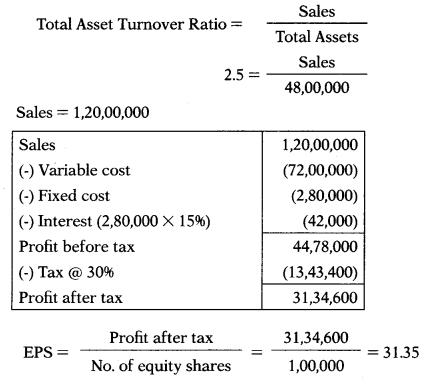Question 173.
Dec 2016: The relevant data from financial statements of Ross Ltd. as on 31st March, 2016 is given below:
Cash — 1,50,000
Investment (short-term) — 3,30,000
Stock — 25,00,000
Prepaid expenses — 50,000
Current liabilities — 10,00,000
The quick ratio will be —
(A) 0.88:1
(B) 0.93 : 1
(C) 3.43 : 1
(D) 3.1 : 1
(A) 0.88:1
Quick ratio$$\frac{\text { Liquid assets }}{\text { Current liabilities }}=\frac{8,80,000}{10,00,000}$$ = 0.88

Question 174.
Dec 2016: From the books of Raja & Co., following details as on 31st March, 2016 are collected:
Equity share capital — 20,00,000
Retained earnings — 10,00,000
1096 Debentures — 20,00,000
Current liabilities — 10,00,000
Profit before interest & tax — 12,00,000
Interest — 1,60,000
Tax — 3,12,000
The rate of return on capital employed will be —
(A) 3096
(B) 2496
(C) 14.56%
(D) 17.76%
(B) 2496
Capital employed = 20,00,000 + 10,00,000 + 20,00,000 = 50,00,000
Return on capital employed = $$=\frac{\text { Operating profit }}{\text { ‘Capital employed }} \times 100=\frac{12,00,000}{50,00,000} \times 100=24 \%$$

Question 175.
Dec 2016: The net profit margin of Rose Ltd. is 896, its total assets are ₹ 6,00,000 and the return on investment is 1896. Total assets turnover will be —
(A) 2.05
(B) 3.15
(C) 2.25
(D) None of the above
(C) 2.25
Total assets turnover =$$\frac{18}{8}$$= 2.25

Question 176.
Dec 2016: Which of the following is not a limitation of financial statements —
(A) Financial statements are essentially interim reports and therefore, cannot be final because the final gain or loss can be computed only at the termination of the business
(B) The values ascribed to the assets presented in the statements depend upon the standards of the person dealing with them
(C) Financial statements fail to bring out the significance of non-financial factors
(D) Financial statements serve as a useful guide for the stakeholders of the company.
(D) Financial statements serve as a useful guide for the stakeholders of the company.

Question 177.
Dec 2016: A company has annual sales of ₹ 150 lakh entirely on credit. It keeps an average inventory sufficient to meet sales demand for half a month and gives its customers one month credit. Its average current liabilities are ₹ 10 lakh. The company must maintain cash and bank balance to have current ratio of 2. The amount of cash balance will be —
(A) ₹ 1,25,000
(B) ₹ 3,00,000
(C) ₹ 13,75,000
(D) ₹ 7,50,000
(A) ₹ 1,25,000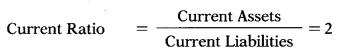Current Assets = 20,00,000
Inventory = 1,50,00,0000 × 0.5/12 = 6,25,000
(valued at sale price in absence of information about cost)
Debtors = 1,50,00,0000 × 1/12 = 12,50,000
Cash balance = 20,00,000 – 6,25,000 – 12,50,000 = 1,25,000

Question 178.
Dec 2016:
Assertion (A)
Accountants do not take into consideration the price level changes while valuing various assets in different period.
Reason (R)
It is difficult to determine the value of assets, as value of assets changes with change in time.
Select the correct answer from the options given below —
(A) Both A and R are true and R is the correct explanation of A
(B) Both A and R are true but R is not the correct explanation of A
(C) A is true, but R is false
(D) A is false, but R is true
(A) Both A and R are true and R is the correct explanation of A

Question 179.
Dec 2016: Gross profit ratio for a firm was 2096 in the year 2015 and 2016 but the net profit ratio was 1596 in the year 2015 and 1296 in the year 2016. The reason for such behaviour could be —
(A) Increase in manufacturing expenses
(B) Increase in indirect expenses
(C) Increase in cost of goods sold
(D) Decrease in sales
(B) Increase in indirect expenses

Question 180.
Dec 2016: The capital of Juhi Ltd. is as follows:
10% Preference shares of ₹ 10 each — ₹ 5,00,000
Equity shares of ₹ 100 each — ₹ 7,00,000
Profit (after tax @ 50%) — ₹ 1,55,000
Depreciation — ₹ 60,000
P/E ratio — 12 times
The market price of equity share will be
(A) ₹ 265.71
(B) ₹ 162.86
(C) ₹ 180
(D) ₹ 156
(C) ₹ 180Question 181.
Dec 2016: Match the following:
List -I — List – II
(P) The standard ratio 2:1 is considered satisfactory — (1) Return on assets
(Q) It measures profitability of the firm in terms of assets employed — (2) Current ratio
(R) Ratio which measures long-term solvency of a firm — (3) Gearing ratio
(S) Ratio which indicates how much of the business is funded by borrowings — (4) Debt-equity ratio
Select the correct answer from the options given below —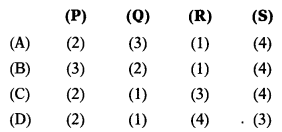(C)

Question 182.
Dec 2016: Total sales:₹ 24,00,000; Inventory turnover: 4.80 times on basis of cost ratio of goods sold; Gross profit ratio: 25% on cost of goods sold; Closing inventory is ₹ 60,000 more than opening inventory. The amount of opening stock and purchases respectively will be —
(A) ₹ 3,70,000 & ₹ 19,80,000
(B) ₹ 3,45,000 & ₹ 18,60,000
(C) ₹ 3,75,000 & ₹ 19,20,000
(D) None of the above
(A) ₹ 3,70,000 & ₹ 19,80,000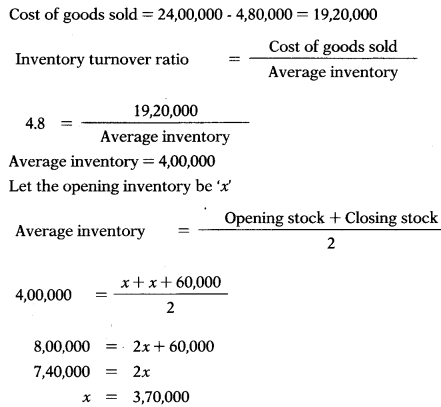Opening stock + Purchases – Closing stock = Cost of goods sold
3,70,000 + Purchases – 4,30,000 = 19,20,000
Purchases = 19,80,000

Question 183.
Dec 2016:
Statement -I
Working capital is a short-term capital which is financed from long-term sources.
Statement – II
Working capital turnover measures the relationship of working capital with sales.
Select the correct answer from the options given below —
(A) Both statements are correct
(B) Both statements are incorrect
(C) Statement-I is incorrect, but Statement-II is correct
(D) Statement-I is correct, but Statement-II is incorrect
(C) Statement-I is incorrect, but Statement-II is correct

Question 184.
Dec 2016:
Owners equity : — ₹ 1,00,000
Current debt to total debt : — 0.40
Total debt to owners’ equity : — 0.60
Fixed assets to owners’ equity : — 0.60
Total assets turnover : — 2 times
Inventory turnover : — 8 times
Fixed assets will be —
(A) ₹ 70,000
(B) ₹ 60,000
(C) ₹ 65,000
(D) ₹ 72,000
(B) ₹ 60,000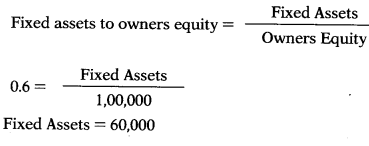Question 185.
June 2017: Fill in the blank space.
Dividend per share = $$\frac{\text { Dividend per share }}{\text { Market price per share }} \times 100=$$
(A) Payout ratio
(B) Earning yield ratio
(C) Dividend yield ratio
(D) Dividend ratio
(C) Dividend yield ratio

Question 186.
June 2017: Proprietor’s net capital employed is known as:
(A) Net Worth
(B) Equity shares
(C) Long-term loans
(D) Fixed assets
(A) Net Worth

Question 187.
June 2017: EBIT/Total assets ratio is:
(A) Liquidity ratio
(B) Profitability ratio
(C) Solvency ratio
(D) Turnover ratio
(B) Profitability ratio

Question 188.
June 2017: Fill in the blank space.
$$\frac{\text { Cost of Sales }+\text { Operating Exp. }}{\text { Sales }} \times 100=\ldots$$
(A) Sales ratio
(B) Sales operating ratio
(C) Operating ratio
(D) Cost sales ratio
(C) Operating ratio

Question 189.
June 2017: Credit sales ₹ 3,00,000, Opening balance of accounts receivable ₹ 50,000 and Closing balance of accounts receivable ₹ 70,000 (assuming 360 days in a year). Debtors turnover ratio will be:
(A) 5
(B) 6
(C) 4
(D) 7
(A) 5Question 190.
June 2017: Short-term solvency is indicated by:
(A) Debtors turnover ratio
(B) Liquid ratio
(C) Price earning ratio
(D) Stock turnover ratio
(B) Liquid ratio

Question 191.
June 2017: Match the following:
List – I ———— List – II
(a) Prepaid expenses — (1) Solvency ratio
(b) Sales ratio — (2) Net profit margin × investment ratio
(c) Return on investment — (3) Turnover ratio
(d) 100-Propritary ratio — (4) Current assets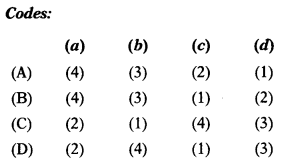(A)

Question 192.
June 2018: The ratio that explains how efficiently companies use their assets to generate sales is:
(A) Revenue asset ratio
(B) Receivable turnover ratio
(C) Income ratio
(D) Fixed Asset turnover ratio
(D) Fixed Asset turnover ratio

Question 193.
June 2018: Profit before interest and tax of AB Ltd. was ₹ 3,40,000. Their net fixed assets were ₹ 2,10,00,000 and working capital was ₹ 27,70,000. Return on investments = ?
(A) 1.3496
(B) 0.5796
(C) 1.4396
(D) 1.5796
(C) 1.4396
Capital Employed = 2,10,00,000 + 27,70,000 = 2,37,70,000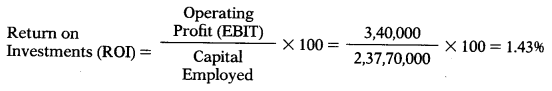Question 194.
June 2018: Debtor velocity = 3 months; Sales ₹ 25,00,000; Bills receivable & bills payable were ₹ 60,000 and ₹ 36,667 respectively. Sundry debtors = ?
(A) ₹ 6,25,000
(B) ₹ 5,25,000
(C) ₹ 5,65,000
(D) ₹ 6,65,000
(C) ₹ 5,65,000
$$3=\frac{x}{25,00,000} \times 12$$
x = Account receivable = 6,25,000
Debtors + Bills receivable = Account receivable
x + 60,000 = 6,25,000
x = Debtors = 5,65,000

Question 195.
June 2018: Working capital ratio is also known as:
(A) Quick ratio
(B) Current ratio
(C) Debt equity ratio
(D) Liquidity ratio
(B) Current ratio

Question 196.
June 2018: For the financial year ended 31st March, 2017, the figures extracted from the balance sheet of EXE Ltd. are as follow:
Opening stock —
Closing stock — 31,000
Cost of goods sold — 2,40,000
The stock turnover ratio will be:
(A) 12 times
(B) 10 times
(C) 8 times
(D) 9 times
(C) 8 timesQuestion 197.
June 2018: Debt service coverage ratio is obtained by dividing net profit before interest and taxes by:
(A) Taxes
(B) Income
(C) Equity
(D) Interest charges
(D) Interest charges

Question 198.
June 2018: Working capital will not change if there is:
(A) Increase in current assets
(B) Payment to the creditors
(C) Decrease in current liabilities
(D) Decrease in current asset
(B) Payment to the creditors

Question 199.
June 2018: Long term solvency is indicated by:
(A) Debt equity ratio
(B) Proprietary ratio
(C) Fixed assets ratio
(D) All of the above
(D) All of the above

Question 200.
Dec2018: Which of the following is not an objective of Management Accounting
(A) To formulate planning and policy
(B) To provide report
(C) To determine the selling price
(D) To assist in decision-making process
(C) To determine the selling price

Question 201.
Dec 2018: If Working Capital is ₹ 24 Lakh, Total Debt is₹ 52 Lakh and Long-term Debt is ₹ 40 Lakh, then current ratio will be:
(A) 2:1
(B) 3:1
(C) 0.6:1
(D) 1.9:1
(B) 3:1
Current liability = 52 – 40 = 12 lakh; Current assets = 24 + 12 = 36 lakh
Current ratio = $$\frac{36}{12}=3$$

Question 202.
Dec 2018: If Stock, Current Assets and Working Capital are ₹ 25 lakh, ₹ 80 lakh and ₹ 30 lakh respectively, then liquid ratio will be:
(A) 2.67:1
(B) 1.45:1
(C) 1.83:1
(D) 1.1:1
(D) 1.1:1
Working capital = Current Assets – Current liabilities
30 lakh = 80 lakh – Current liabilities
Current liabilities = 50 lakh
Liquid assets = 80 lakh – 25 lakh = 55 lakh
Current ratio = $$\frac{55}{50}=1.1$$

Question 203.
Dec 2018: If current ratio is 2.5:1 and Working Capital is ₹ 120 Lakh, then current liabilities are:
(A) ₹ 48 Lakh
(B) ₹ 200 Lakh
(C) ₹ 80 Lakh
(D) ₹ 180 Lakh
(C) ₹ 80 Lakh$$2.5=\frac{x}{y}$$
Working capital = Current Assets – Current liabilities
120 lakh = 2.5y – y
120 lakh = 1.5 y
y = Current liabilities = 80 lakh

Question 204.
Dec 2018: If Average Inventory is ₹ 125 Lakh, Inventory Turnover Ratio is 8 times and Profit is 20% on sales, then amount of sales will be:
(A) ₹ 1,000 Lakh
(B) ₹ 1,200 Lakh
(C) ₹ 800 Lakh
(D) ₹ 1,250 Lakh
(D) ₹ 1,250 LakhCost of goods sold = 1,000 lakh
Sales = 1,000 lakh × 125% = 1250 lakh

Question 205.
Dec 2018: Current Ratio is 2.5:1 and Liquid Ratio is 1.5:1. If inventory is ₹ 9,60,000, then the amount of current assets will be:
(A) ₹ 9.6 Lakh
(B) ₹ 14.40 Lakh
(C) ₹ 24 Lakh
(D) ₹ 38.40 Lakh
(C) ₹ 24 Lakh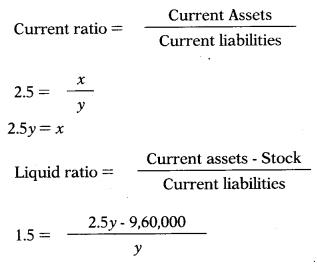1.5y =2.5y- 9,60,000
y = 9,60,000
x = 9,60,000 × 2.5 = 24,00,000

Question 206.
Dec 2018: Cost of Goods Sold is ₹ 90 Lakh, Purchases are ₹ 96 Lakh and Closing Stock is ₹ 18 Lakh, then Stock Turnover Ratio will be:
(A) 5 times
(B) 6 times
(C) 6.4 times
(D) 4.29 times
(A) 5 times

Question 207.
Dec 2018: If Market Price per share, Earning per share and Dividend per share are ₹ 150, ₹ 16.50 and ₹ 15 respectively, then Price Earnings Ratio will be:
(A) 10 times
(B) 9.09 times
(C) 1.1 times
(D) 0.91 times
(B) 9.09 times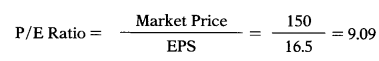Question 208.
Dec 2018: Capital Gearing Ratio is categorized as:
(A) Profitability Ratio
(B) Activity Ratio
(C) Long-term Solvency Ratio
(D) Market Test Ratio
(C) Long-term Solvency Ratio

Question 209.
June 2019: Dividing net credit sales by average debtors would yield
(A) Current ratio
(B) Return on sales ratio
(C) Debtors turnover ratio
(D) Average receivables
(C) Debtors turnover ratio

Question 210.
June 2019: ABC Ltd. has earned 12% returns on total assets of ₹ 8,00,000 and has a net profit ratio of 8%. Sales of the firm shall be:
(A) ₹ 96,000
(B) ₹ 6,40,000
(C) ₹ 12,00,000
(D) ₹ 7,36,000
(C) ₹ 12,00,000

Question 211.
June 2019: 9% preference shares of ₹ 10 each ₹ 4,00,000, Equity shares of ₹ 10 each ₹ 12,00,000, Profit after tax ₹ 4,20,000, Equity dividend paid 20%, Market price of equity shares ₹ 25 each. What will be the earnings per share
(A) 3.50
(B) 3.20
(C) 5.40
(D) 9.60
(B) 3.20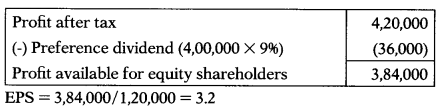Question 212.
June 2019: The average creditors are ₹ 74,000, creditors turnover ratio is 4.80. amount of credit purchase will be:
(A) ₹ 15,417
(B) ₹ 3,52,500
(C) ₹ 3,55,200
(D) None of the above
(C) ₹ 3,55,200
74,000 × 4.8 = 3,55,200

Question 213.
June 2019: What will be the amount of stock if the current ratio is 2:1 and quick ratio is 1.5:1 and current liabilities are ₹ 90,000
(A) ₹ 55,000
(B) ₹ 1,80,000
(C) ₹ 1,35,000
(D) ₹ 45,000
(D) ₹ 45,000Question 214.
June 2019: Conducted to ensure borrowings capacity of a concern to meet contingencies in near future is:
(A) Long term analysis
(B) Vertical analysis
(C) Short term analysis
(D) Internal analysis
(C) Short term analysis

Question 215.
June 2019: A company has an inventory of ₹ 58,400, Debtors of ₹ 48,000 and inventory turnover 6 times. The gross profit margin is 20% on sales and its credit sales are 40% of the total sales. What will be the credit sales
(A) ₹ 3,50,400
(B) ₹ 3,53,440
(C) ₹ 4,38,000
(D) ₹ 1,75,200
(D) ₹ 1,75,200
Cost of goods sold = 58,400 × 6 = 3,50,400
GP ratio is 20% on sales that mean it is 25% on cost.
Sales = 3,50,000 + 87,600 = 4,38,000
Credit sales = 4,38,000 × 40% = 1,75,200

Question 216.
June 2019: The following information is given for X Ltd.:
Stock velocity 3.75 months. Gross profit ₹ 80,000 being 20% of sales. The closing stock of the year is ₹ 25,000 more than opening stock. What will be the amount of opening stock and closing stock
(A) ₹ 1,00,000 and ₹ 1,25,000
(B) ₹ 80,000 and ₹ 1,05,000
(C) ₹ 87,500and ₹ 1,12,500
(D) ₹ 85,000 and ₹ 1,10,000
(C) ₹ 87,500 and ₹ 1,12,500
Sales = 80,000/20% = 4,00,000
Cost of goods sold = 4,00,000 – 80,000 = 3,20,000
Opening Stock = x
Closing Stock = x + 25,000
Average Stock = (x + x + 25,000)/2
Average Stock = (2x + 25,000)/2
Average Stock = x + 12,50012,00,000 = 12x+ 1,50,000
x = Opening Stock = 87,500
Closing Stock = 87,500 + 25,000 = 1,12,500

Question 217.
June 2019: A company has the following Current Assets:
Cash — ₹ 40,000
Marketable securities — ₹ 25,000
Debtors — ₹20,000
Inventory — ₹18,000
Total current liabilities were ₹ 65,400 (including the future tax liability of ₹ 4,800 which will be made after one year). What will be the quick ratio of the company
(A) 1.40:1
(B) 1.81:1
(C) 1.57:1
(D) 1.30:1
(A) 1.40:1
Quick Ratio = 40,000 + 25,000 + 20,000/65,400 – 4,800 = 85,000/60,600=1.40

Question 218.
June 2019: Closing debtors are ₹ 8,00,000which are 125 percent of opening debtors. Cash sales are 25 percent of total sales. If the debtor’s turnover ratio is 4 times then the amount of total sales will be ………………
(A) ₹ 36,00,000
(B) ₹ 28,80,000
(C) ₹ 38,40,000
(D) ₹ 48,00,000